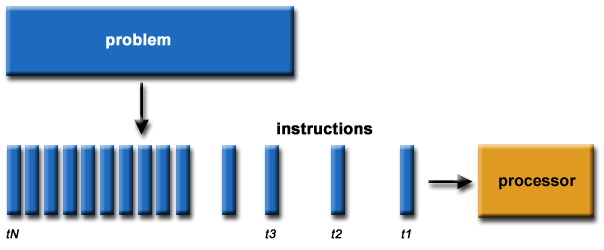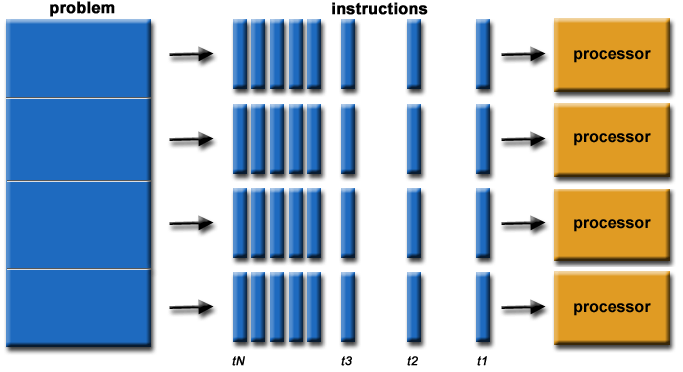Using Parallel Computing to Speed-up Python & R Codes

## Introduction

Most modern computers are equipped with multiple Central Processing Units, while most people are still used to writing codes in a serial-computing fashion which only makes use of a single processor at a time.

Example 1: We need to extract two big-size tables, table A and table B, from a remote database. These two extractions are independent. People normally extract one of them first and only start another extraction after the first one is done. Most of the time will be spent waiting for network I/O and disk I/O.

Example 2: You may need to apply a function on each element of a long array (say containing a few million elements) which may take quite long time. You may notice your CPU usage keeps at about 25%, which means 3 out of 4 CPU cores you have are idle while you’re waiting for the calculation results.

This results in inefficient usage of your computing power. This concern may sound only applicable for large-scale computing on supercomputers. However it’s not. You can do parallel computing on your laptop too, using multiprocessing technique.

In my daily works, I found this may accelerate my scripts by up to 60%, which is quite considerable.

Serial ComputingParallel Computing## multiprocessing in Python

We normally use module multiprocessing to do single-machine parallel computing in Python. It supports spawning processes using an API similar to the threading module. It uses subprocesses to side-step GIL, therefore allowing us fully leverage multiple processors on our machine .

Let’s have a look at the pseudo code solving example-1.

from multiprocessing import Process

def FUN_extract_table_1(args):
# connect with romote database
# extract table
# write to local disk
pass
def FUN_extract_table_2(args):
# connect with romote database
# extract table
# write to local disk
pass

if __name__ == "__main__":
# Start the processes
paralleled_jobs = []

arguments=[]
p = Process(target=FUN_extract_table_1, args=(arguments,))
p.start()
paralleled_jobs.append(p)

p = Process(target=FUN_extract_table_2, args=(arguments,))
p.start()
paralleled_jobs.append(p)

# Join the processes, to block the calling thread until the process whose "join()" method is called terminates or until the optional timeout occurs
for p in paralleled_jobs:
p.join()

The two tasks, FUN_extract_table_1 and FUN_extract_table_1, will be run concurrently. If they take 5 minutes and 4 minutes separately, serial computing will take 9 minutes in total while parallel computing may only take 5 to 6 minutes (for local CPU-intensive tasks, it may not be as efficient as this).

Normally we decide how many concurrent tasks we start based on how many CPU cores we have (can be obtained using multiprocessing.cpu_count()). But in settings like example-1, actually we can have more concurrent processes. This is because the tasks in example-1 are not local CPU-intensive or local RAM-intensive. The main pressure comes from network I/O, which will not be significantly affected by the number of concurrent local processes.

In local computing-intensive tasks, we should be careful about how many concurrent processes we have. And the acceleration factor may be smaller. If we split a task into two sub-tasks and assign them to two processing units, we can’t expect the running time to be halved (this is well explained by Amdahl’s law).

There are some other key parts we need to take into consideration when we design a parallel computing process:

• If multiple processes output to the same object at the same time, there may be conflict or even error. We can use a Lock to ensure only one process can output to a specific object at a time (please refer to Synchronization between processes).

• We may need to exchange information between processes, or collect results from sub-processes. In multiprocessing, there are a few ways to tackle this, including Pipe, Queue (reference), and Manager (reference).

• We also need to ensure the work loads among processes are balanced. The reason behind is quite straightforward: imagine you give all hard works to worker A and all easy works to worker B. Even if the number of tasks are equal, worker A may be occupied for the whole day while worker B is idle for most of the time.

• If the tasks we’re trying to parallelize are dependent on each other, we need to be more careful when we design the process and implement. If they’re not, lucky you ;-).

I have prepared another sample code below, in which we use two processors concurrently to calculate the sum of a sequence and compare the result & lapse time with another single-processing method. We use Manager for inter-process communication.

import time
from multiprocessing import Process, Manager

def FUN_test(vector_to_use, list_to_dump_result):
temp = 0
for i in vector_to_use:
temp += (i+1)

if list_to_dump_result != None:
list_to_dump_result.append(temp)
else:
print("Single: "+ str(temp))

length = int(99999999)

if __name__ == '__main__':

time_1 = time.time()
# Single-process computing
FUN_test(xrange(length), None)

time_2 = time.time()
# split the data so that it can be processed in parallel style
cut_point = int(length/2)
data_to_parallel = [xrange(cut_point), xrange(cut_point, length)]

# Build a manager for inter-process communication
manager = Manager()
list_of_result = manager.list()

# Start the processes
paralleled_jobs = []

for data in data_to_parallel:
p = Process(target=FUN_test, args=(data, list_of_result, ))
p.start()
paralleled_jobs.append(p)
print("{}(pid {}) is started.".format(p.name, p.pid))

# Join the processes, to block the calling thread until the process whose "join()" method is called terminates or until the optional timeout occurs
for p in paralleled_jobs:
p.join()
print("{}(pid {}) is joined.".format(p.name, p.pid))

# Collect the results
print("Parallel Result: {}".format(sum(list_of_result)))

time_3 = time.time()

# Report the lapse difference between the two methods
laspse_single_proc = time_2 - time_1
laspse_multi_proc = time_3 - time_2
print("Single Processing Lapse: {}".format(laspse_single_proc))
print("Multiple Processing Lapse: {}".format(laspse_multi_proc))
print("Acceleration Factor: {}".format(laspse_single_proc/laspse_multi_proc))
## Single: 4999999950000000
## Process-2(pid 1627) is started.
## Process-3(pid 1628) is started.
## Process-2(pid 1627) is joined.
## Process-3(pid 1628) is joined.
## Parallel Result: 4999999950000000
## Single Processing Lapse: 5.02888607979
## Multiple Processing Lapse: 2.49400997162
## Acceleration Factor: 2.01638571498

(Note: the code above is written in Python 2.7. To run it under Python 3, the only change needed is to replace xrange with range.)

## snowfall in R

There are multiple packges supporting (local) parallel computing in R language, including parallel, snowfall, foreach, etc. Here I would like to introduce snowfall only, as its API design allows seamless integrating with “normal” R codes. Experienced R users are normally familiar with the *apply function family, like sapply. With snowfall, the only change is to replace sapply with sfSapply (after initializing your local cluster). Can’t be easier!

rm(list=ls())
library(microbenchmark)
library(snowfall)  # dependes on package "snow"
## Loading required package: snow
# define a toy function for testing
calcPar <- function(x) {
set.seed(123) # set the seed so that we can check if different methods return the same results

x1 <- matrix(0, x, x)
x2 <- matrix(0, x, x)

for(var in 1:nrow(x1))
x1[var,] = runif(ncol(x1))
for(var in 1:nrow(x2))
x2[var,] = runif(ncol(x1))

b <- sum(diag((x1 %*% x2) %*% x1))
return(b)
}

index_to_run <- rep(200, 50)  # The 2nd argument is the length of the 'index_to_run'

# =========================================================================
# 'snowfall' package ------------------------------------------------------

# initialize the cluster
# parallel::detectCores() returns the # of available processors
sfInit(parallel=TRUE, cpus=parallel::detectCores()) 
## Warning in searchCommandline(parallel, cpus = cpus, type = type,
## socketHosts = socketHosts, : Unknown option on commandline:
## rmarkdown::render('/Users/XD/Desktop/Multiprocessing.Rmd',~+~~+~encoding~+~
## R Version:  R version 3.2.3 (2015-12-10)
## snowfall 1.84-6.1 initialized (using snow 0.4-1): parallel execution on 4 CPUs.
# sfClusterApplyLB means "load balance".
# It can help balance the load on each node if the capability of the nodes are different
benchmark.result.snowfall <- microbenchmark(result.1 <- sapply(index_to_run, calcPar),
result.2 <- unlist(sfClusterApplyLB(index_to_run, calcPar)),
result.3 <- sfSapply(index_to_run, calcPar),
times = 5)
# shut off the cluster
sfStop()
##
## Stopping cluster
if(identical(result.1, result.2) && identical(result.1, result.3)){
cat("The calculation results can match.")
} else {
cat("The calculation results can NOT match.")
}
## The calculation results can match.
print(benchmark.result.snowfall)
## Unit: seconds
##                                                         expr      min
##                    result.1 <- sapply(index_to_run, calcPar) 2.669098
##  result.2 <- unlist(sfClusterApplyLB(index_to_run, calcPar)) 1.292744
##                  result.3 <- sfSapply(index_to_run, calcPar) 1.164066
##        lq     mean   median       uq      max neval
##  2.694608 2.696824 2.695723 2.697860 2.726830     5
##  1.318452 1.317806 1.322544 1.325614 1.329677     5
##  1.187064 1.197022 1.191988 1.207601 1.234391     5

As we can see, the results are all identical, while parallel-computing codes consume about half of the time required by the serial-computing code.

We need to note that if our computation requires additional data other than those passed as arguments, we need to export the required data to each node with function sfExport(). Otherwise we will encounter errors. However, this sort of operations may also introduce heavier overhead.